#### 3.1.2Galerkin’s Method

The Galerkin method was developed by the mathematicians Boris Galerkin and Walther Ritz (separately) to treat variational problems similar to (3.4) . In fact, Galerkin attempted to solve a restricted version of (3.4) as described by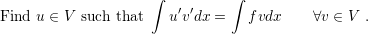(3.5)

The constraints of the solutionto the same space of the test function () is very convenient, as it will be shown in the end of Section 3.1.5, but the central question right now is, if the solutionof (3.5) still satisfies the original PDE, (3.1). In order to prove that it does, a good strategy is to derive (3.1) from (3.5). Hence, letbe the solution of (3.5), then it follows that (3.6) must also be satisfied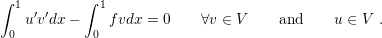(3.6)

In addition to the restrictions imposed on, consider that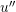exists and is continuous. Integrating the first term by parts and using the boundary conditions (v(0) = v(1) = 0) the following relation holds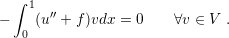(3.7)

Since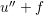is continuous, (3.7) can only stand, if the following relation is true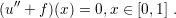(3.8)

Consequently,satisfies the original BVP (3.1). However, it was necessary to assumeas a well behaved function (second differentiable continuous). This restriction should satisfy most physical problems, but attention must be paid for careless application of Galerkin’s method.

So far only the equivalence of the solution between Galerkin’s problem and (3.1) was discussed, but not the method itself. Galerkin’s method is developed upon the discretization of (3.6) based on the discretization of the space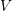as presented in the next section.